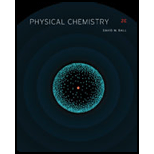# Use the conditions of exercise 19.12 to determine the mean free path between hydrogen atoms in interstellar space if d = 1.10 A ∘ for hydrogen.### Physical Chemistry

2nd Edition
Ball + 3 others
ISBN: 9781133958437

#### Solutions

Chapter
Section### Physical Chemistry

2nd Edition
Ball + 3 others
ISBN: 9781133958437
Chapter 19, Problem 19.39E
Textbook Problem
15 views

## Use the conditions of exercise 19.12 to determine the mean free path between hydrogen atoms in interstellar space if d = 1.10 A ∘ for hydrogen.

Interpretation Introduction

Interpretation:

The mean free path between hydrogen atoms in interstellar space if d=1.10A for hydrogen is to be calculated by using the conditions of exercise 19.12.

Concept introduction:

The mean free path is calculated by the formula,

λ=V2πNd2

Where,

V is the volume of the gas.

N is the number of atoms.

d is the interstellar space.

### Explanation of Solution

The mean free path is calculated by the formula,

λ=V2πNd2 …(1)

The volume of gas is 106m3. The number of atoms present in 1cm3 is 10.

Substitute the volume and number of atoms in equation (1).

λ=106m32×3

### Still sussing out bartleby?

Check out a sample textbook solution.

See a sample solution

#### The Solution to Your Study Problems

Bartleby provides explanations to thousands of textbook problems written by our experts, many with advanced degrees!

Get Started

Find more solutions based on key concepts
Which of the following statements about basal metabolic rate (BMR) is correct? The greater a person's ago, the ...

Nutrition: Concepts and Controversies - Standalone book (MindTap Course List)

25-6 What are the components of (a) a nucleotide and (b) a nucleoside?

Introduction to General, Organic and Biochemistry

Identify the trends in electronegativities in the periodic table.

Introductory Chemistry: An Active Learning Approach

Why is there a main sequence?

Foundations of Astronomy (MindTap Course List)

The distance between the centers of the wheels of a motorcycle is 155 cm. The center of mass of the motorcycle,...

Physics for Scientists and Engineers, Technology Update (No access codes included)